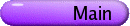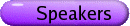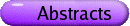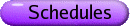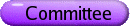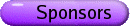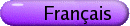Operator Algebras Org: Marcelo Laca and John Phillips (Victoria)[PDF] BEATRIZ ABADIE, Universidad de la Republica, Montevideo, Uruguay Morita equivalence for Quantum Heisenberg Manifolds [PDF] Quantum Heisenberg manifolds were constructed by M. Rieffel as a quantization deformation of certain homogeneous spaces H/Nc, H being the Heisenberg group. We describe Morita equivalence within this family by adapting to this setting some of the techniques employed in the analogous discussion for non-commutative tori and Heisenberg C*-algebras. One of the main tools employed is the generalization of a result of P. Green and M. Rieffel about Morita equivalence of transformation group C*-algebras corresponding to two commuting free and proper actions on a topological space; this result is generalized to the context of crossed products by Hilbert C*-bimodules. BERNDT BRENKEN, University of Calgary The isolated ideal of a correspondence associated with a topological quiver [PDF] For a general C*-correspondence over a C*-algebra A we identify a hereditary saturated ideal of isolated points of A. The Cuntz-Pimsner C*-algebra of the quotient correspondence is both a relative Cuntz-Pimsner algebra and a quotient of the Cuntz-Pimsner algebra of the original correspondence. For the C*-correspondence arising from a topological quiver one obtains a correspondence associated with the restriction of the quiver to the non-isolated vertices. The restricted topological quiver satisfies conditions (L) or (K) if and only if the original quiver does. KEN DAVIDSON, University of Waterloo Nest representations of directed graph algebras [PDF] To each directed graph, there are two natural non-self-adjoint operator algebras. We investigate nest representations of these algebras, i.e., representations with range that is dense in an algebra of upper triangular matrices or a nest algebra. In particular, we show that the finite dimensional nest representations separate points and we characterize when there is a faithful nest representation. This is joint work with Elias Katsoulis. GEORGE ELLIOTT, University of Toronto, Toronto, Ontario M5S 2E4 On the classification of non-simple inductive limits of matrix algebras over the interval-the theorem of Robert [PDF] An outline is given of the statement and proof of the theorem of Leonel Robert the brief statement of which is as follows: The inductive limits of matrix algebras over the interval are classified by certain elementary K-theoretical invariants (including traces). (At the date of writing, the statement is restricted to the case that the set of closed two-sided ideals of the inductive limit C*-algebra is totally ordered.) HEATH EMERSON, University of Victoria A Gysin sequence in noncommutative geometry [PDF] For a class of group actions on compact spaces, we construct an analogue of the Gysin sequence in classical topology (which computes the map on cohomology induced by the projection from the sphere bundle of a compact manifold, to the manifold). The noncommutative sequence computes the map on K-theory induced by the inclusion of the reduced C*-algebra of G into the cross-product C(X) ×G. Applications are given to a problem in index theory: namely, we give a KK-theoretic proof of the theorem of Luck and Rosenberg identifying the class of the de Rham operator in equivariant KK, with the class of a certain combinatorial Euler class. THIERRY GIORDANO, University of Ottawa, Ottawa Topological orbit equivalence of free, minimal actions of Z2 on the Cantor set [PDF] In 1959, H. Dye introduced the notion of orbit equivalence and proved that any two ergodic finite measure preserving transformations on a Lebesgue space are orbit equivalent. He also conjectured that an arbitrary action of a discrete amenable group is orbit equivalent to a Z-action. This conjecture was proved by Ornstein and Weiss and its most general case by Connes, Feldman and Weiss by establishing that an amenable non-singular countable equivalence relation R can be generated by a single transformation, or equivalently is hyperfinite, i.e., R is up to a null set, a countable increasing union of finite equivalence relations. In the Borel case, Weiss proved that actions of Zn are (orbit equivalent to) hyperfinite Borel equivalence relations, whose classification was obtained by Dougherty, Jackson and Kechris. In 1995, Giordano, Putnam and Skau proved that minimal Z-actions on the Cantor set were orbit equivalent to approximately finite (AF) relations and their classification was given. Since then some special classes of minimal free actions of Z2 on the Cantor set were shown to be affable (i.e., orbit equivalent to AF-relations). In this talk I will indicate the main steps of the proof of the general result obtained in a joint effort with H. Matui, I. Putnam and C. Skau and whose statement is the following: Theorem: Any minimal, free Z2-action on the Cantor set is affable. DANIEL GONCALVES, University of Victoria An Introduction to New C*-algebras from Tilings [PDF] Given a tiling that satisfies some standard conditions, we introduce an equivalence relation on Rd by saying that two points, x and y, are equivalent if the patch defined by y on the tiling matches the patch defined by x translated by y-x. We then consider the C*-algebra associated to this equivalence relation and describe some of its ideals. If time permits we will show that this is a recursive subhomogeneous C*-algebra. In the case of a substitution tiling we use the inflation map to get an inductive limit of C*-algebras, which is simple. We finish with a few K-theory computations for some examples. CRISTIAN IVANESCU, University of Northern British Columbia Continuous trace C*-algebras with spectrum [0,1], inductive limits of continuous trace C*-algebras and their classification [PDF] A classification result will be presented for the class of simple separable C*-algebras which are inductive limits of continuous trace C*-algebras with spectrum [0,1] or finitely many copies of such a space. An important step that will be discussed is the pulling back of the invariant from the inductive limit stage to the finite stage. As will be explained, a new technique is necessary, namely the use of what we call "a gap" interpreted in a suitable sense. Joint work with George A. Elliott. DAVID KERR, Texas A&M University, College Station, TX, USA 77843-3368 Dynamics and rigidity in simple C*-algebras [PDF] The achievements of the Elliott classification program have established a striking picture of cohomological rigidity within a large class of simple nuclear C*-algebras. I will discuss some consequences of this rigidity for the dynamics of typical automorphisms. CLAUS KOESTLER, Carleton University Central Limit Laws on Jones Towers [PDF] The re-interpretation of commuting squares as a noncommutative version of stochastic independence gives rise to the notion of Bernoulli shifts on Jones towers. We investigate central limit theorems on such towers and show that such laws exist for shifts with a homogeneous product representation. In particular, we report experimental results indicating that Wigner's semicircle law is among them. This is joint work with Rolf Gohm. HANGFENG LI, SUNY at Buffalo, Buffalo, New York A Topology for Ergodic Actions of Compact Quantum Groups [PDF] We show that there is a natural topology on the set of all isomorphism classes of ergodic actions of any fixed compact quantum group. HUAXIN LIN, University of Oregon The Rokhlin property for automorphisms on simple C*-algebras [PDF] We study a general Kishimoto's problem for automorphisms on simple C*-algebras with tracial rank zero. The original problem of Kishimoto is the following: Let A be a unital simple AT-algebra with real rank zero and a is an approximately inner automorphism. Is A\rtimesaZ again a simple AT-algebra? Let A be a unital separable simple C*-algebra, with tracial rank zero and let a be an automorphism. Under the assumption that a has certain Rokhlin property, A\rtimesaZ has tracial rank zero. We also show that if the induced map a*0 on K0(A) fixes a "dense" subgroup of K0(A) then the tracial Rokhlin property implies a stronger Rokhlin property. Consequently, the induced crossed product C*-algebras have tracial rank zero. By applying the classification theorem, this answers affirmatively Kishimoto's original question. JAMIE MINGO, Queen's University Second Order Freeness and Compound Wishart Matrices [PDF] In 1986, D. Voiculescu introduced the R-transform of a random variable. The coefficients of this power series, now called free cumulants, were shown in 1994 by R. Speicher to have a combinatorial interpretation using non-crossing partitions. In 2004, A. Nica and I showed that the covariance, when suitably magnified, of the traces of some standard families of random matrices has a limiting value that can be interpreted in terms of non-crossing annular partitions. Indeed the order of elements in a block of a partition is now significant and the relevant concept is a non-crossing permutation. These non-crossing annular permutations give rise to second order cumulants which in turn form the basis of second order freeness. We shall give a number of natural examples of families of matrices that exhibit second order freeness. This work was done in collaboration with B. Collins, A. Nica, P. Sniady, and R. Speicher. IAN PUTNAM, University of Victoria Relative K-theory for C*-algebras and applications [PDF] I will give a description for a relative K-theory determined by an inclusion of a pair of C*-algebras. I will describe a result which shows two such pairs have isomorphic relative K-groups. Finally, I will present some examples arising from problems in orbit equivalence of Cantor minimal systems and from projection method tilings. GUNNAR RESTORFF, University of Copenhagen, Denmark Classification of Cuntz-Krieger algebras [PDF] Mikael Rørdam and Danrun Huang initiated the classification of Cuntz-Krieger algebras in the mid-nineties, and, recently, the classification up to stable isomorphism of Cuntz-Krieger algebras (satisfying the condition (II) of Cuntz) was completed. This work raised other new interesting questions. In my talk I will talk about the classification of Cuntz-Krieger algebras, and state the classification theorem. Also, I am planning to comment on some of the questions raised by this classification result. ANA SAVU, University of Northern British Columbia Closed and exact functions in interacting particle systems [PDF] Statistical physics has developed a whole variety of interacting particle systems to explain the transition from microscopic to macroscopic scale. One of the main difficulties in finding the scaling limit of a nongradient particle system is to make rigorous sense of the fluctuation-dissipation equations. As has been shown by Varadhan, the fluctuation-dissipation equation is equivalent to a certain direct sum decomposition of a Hilbert space. For a general vector field we exhibit two Hilbert spaces, namely the space of so called "closed functions" and the space of "exact functions" and we calculate the codimension of the space of "exact functions" inside the space of "closed functions". In particular we provide a new approach based on Fourier analysis for the two known cases, the Glauber field and the second-order Ginzburg-Landau field, and we provide a solution for a new case, the fourth-order Ginzburg-Landau field. The author is grateful to George A. Elliott for many discussions and ideas that led to the completion of this work.